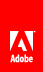Concatenating strings

Flash Player 9 and later, Adobe AIR 1.0 and later

 Concatenation of strings means taking two strings and joining them sequentially into one. For example, you can use the + operator to concatenate two strings: var str1:String = "green"; var str2:String = "ish"; var str3:String = str1 + str2; // str3 == "greenish" You can also use the += operator to the produce the same result, as the following example shows: var str:String = "green"; str += "ish"; // str == "greenish" Additionally, the String class includes a concat() method, which can be used as follows: var str1:String = "Bonjour"; var str2:String = "from"; var str3:String = "Paris"; var str4:String = str1.concat(" ", str2, " ", str3); // str4 == "Bonjour from Paris" If you use the + operator (or the += operator) with a String object and an object that is not a string, ActionScript automatically converts the nonstring object to a String object in order to evaluate the expression, as shown in this example: var str:String = "Area = "; var area:Number = Math.PI * Math.pow(3, 2); str = str + area; // str == "Area = 28.274333882308138" However, you can use parentheses for grouping to provide context for the + operator, as the following example shows: trace("Total: \$" + 4.55 + 1.45); // output: Total: \$4.551.45 trace("Total: \$" + (4.55 + 1.45)); // output: Total: \$6Twitter™ and Facebook posts are not covered under the terms of Creative Commons.// Ethnio survey code removed